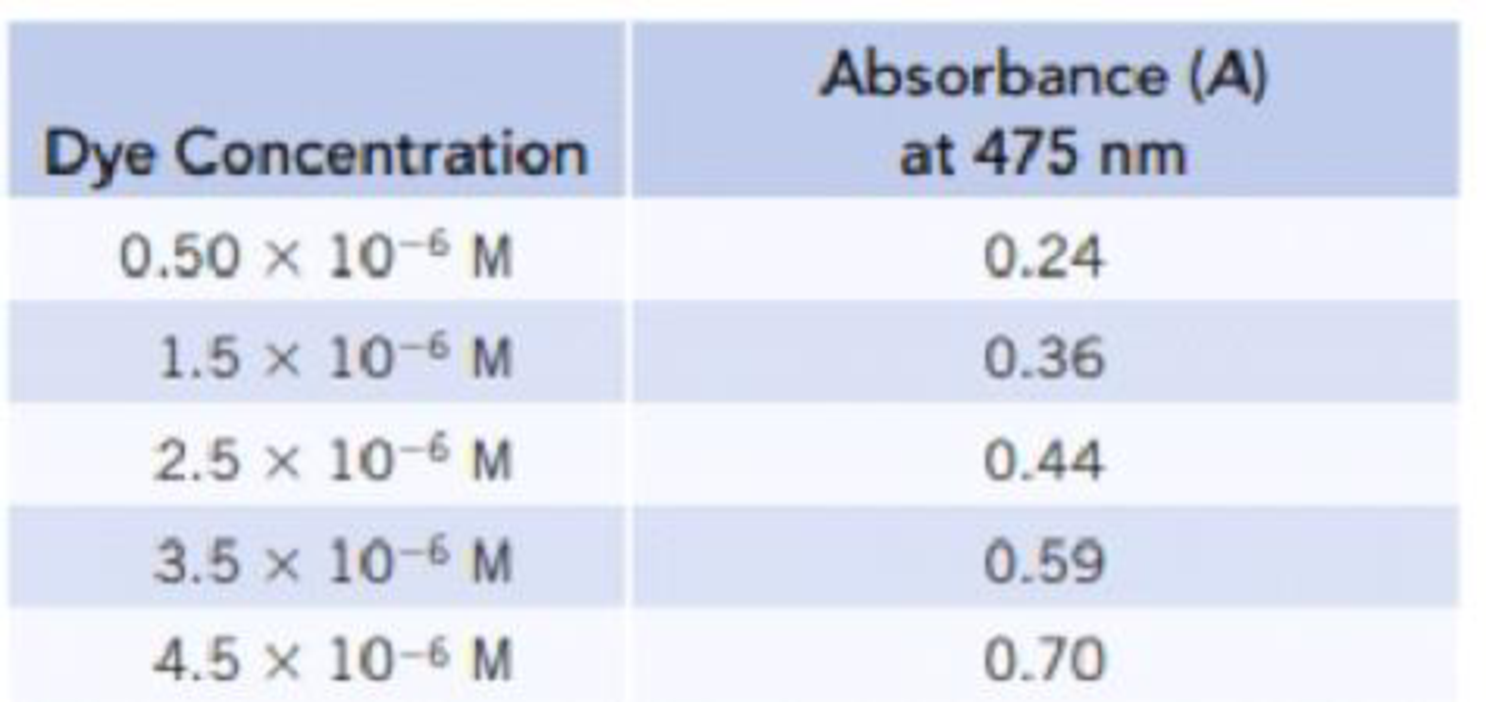# A solution of a dye was analyzed by spectrophotometry, and the following calibration data were collected. (a) Construct a calibration plot, and determine the slope and intercept. (b) What is the dye concentration in a solution with A = 0.52?### Chemistry & Chemical Reactivity

9th Edition
John C. Kotz + 3 others
Publisher: Cengage Learning
ISBN: 9781133949640

#### Solutions

Chapter
Section### Chemistry & Chemical Reactivity

9th Edition
John C. Kotz + 3 others
Publisher: Cengage Learning
ISBN: 9781133949640
Chapter 4, Problem 77PS
Textbook Problem
149 views

## A solution of a dye was analyzed by spectrophotometry, and the following calibration data were collected.(a) Construct a calibration plot, and determine the slope and intercept.(b) What is the dye concentration in a solution with A = 0.52?

(a)

Interpretation Introduction

Interpretation:

By using the given absorbance and concentration values a calibration plot has to be constructed and slope and intercept has to be determined from the calibration plot.

Concept introduction:

• Spectrophotometry is the interaction between light and matter that provides quantitative about solution concentration.
• Absorbance (A): it is the negative logarithm of transmittance of the sample taken. And its value increases as the concentration increases.

Absorbance=-logT=-logPP0

T is the transmittance and it is the ratio of the amount of light transmitted by or passing through the sample (P) relative to the light that initially fell on the sample.

Transmittance(T)=PP0=IntencityoftransmittedlightIntencityofincidentlight

• y=mx+c is a straight line equation where m is the slope and c is the intercept.
• Concentration of the solution can be expressed in many ways; molarity is one of the important parameter to express the concentration of solution. And it is a measure of the concentration of a solute in a solution.

### Explanation of Solution

A calibration plot is constructed by using the given values of dye concentration with the absorbance (A) as follows,

The equation is,

y=mx+c .....

(b)

Interpretation Introduction

Interpretation:

The concentration of the solution with absorbance (A) 0.52  has to be calculated.

Concept introduction:

• Spectrophotometry is the interaction between light and matter that provides quantitative about solution concentration.
• Absorbance (A): it is the negative logarithm of transmittance of the sample taken. And its value increases as the concentration increases.

Absorbance=-logT=-logPP0

T is the transmittance and it is the ratio of the amount of light transmitted by or passing through the sample (P) relative to the light that initially fell on the sample.

Transmittance(T)=PP0=IntencityoftransmittedlightIntencityofincidentlight

• y=mx+c is a straight line equation where m is the slope and c is the intercept.
• Concentration of the solution can be expressed in many ways, molarity is one of the important parameter to express the concentration of solution.  And it is a measure of the concentration of a solute in a solution.

### Still sussing out bartleby?

Check out a sample textbook solution.

See a sample solution

#### The Solution to Your Study Problems

Bartleby provides explanations to thousands of textbook problems written by our experts, many with advanced degrees!

Get Started

Find more solutions based on key concepts
The USDA Eating Patterns recommend a small amount of daily oil from which of these sources? a. olives b. nuts c...

Nutrition: Concepts and Controversies - Standalone book (MindTap Course List)

6.25 Define the term ground state.

Chemistry for Engineering Students

Why did classical astronomers conclude that Earth had to be motionless?

Horizons: Exploring the Universe (MindTap Course List)

A carpenters square has the shape of an L as shown in Figure P12.3. Locate its center of gravity. Figure P12.3

Physics for Scientists and Engineers, Technology Update (No access codes included)

How old is the oldest evidence for life on Earth? On what are those estimates based?

Oceanography: An Invitation To Marine Science, Loose-leaf Versin